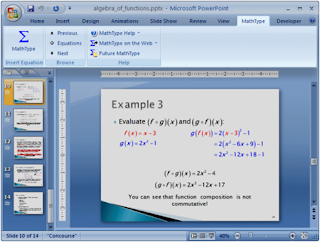## Wednesday, March 16, 2011

### MathType: Equations in PowerPoint

MathType is a powerful interactive equation editor for Windows and Macintosh that lets you create mathematical notation for word processing, web pages, desktop publishing, presentations, elearning, and for TeX, LaTeX, and MathML documents.Photo: Design Science

You've finished your research and you need to present it
You're preparing a lecture using computations and calculations you worked out in various applications. Or say you created a handout for your math class using Word and you want to turn it into a PowerPoint slide. With MathType you can copy equations out of your documents and paste them directly into your presentations.

Reuse existing equations -- save time and avoid mistakes
Instead of creating equations for your slides from scratch, you can save time and avoid mistakes by re-using existing equations. With MathType you can extract equations from hundreds of applications and websites such as Mathematica, Maple, Microsoft Office, Wikipedia, and many more.

4 ways to create new equations
If you don't have existing equations you can reuse, MathType 6.7 offers four approaches to creating equations in your presentations:
• Interactive: Save time by using point-and-click templates and symbols. MathType applies mathematical spacing rules automatically as you type.
• Keyboard shortcuts: Great for those that prefer using the keyboard to create equations. Insert a new equation, or edit an existing one, using just a keystroke.
• TeX/LaTeX input: If you already know the TeX typesetting language, MathType allows you to build equations by typing TeX into MathType's editing window.
• Type TeX/LaTeX directly into Microsoft Word (Windows Only): You can enter equations directly into Word documents. Use the Toggle TeX command (Alt+\) to turn what you typed into a MathType equation. Use it again to edit the TeX language.